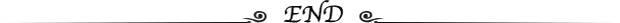# MediaCodec 解码后数据对齐导致的绿边问题

06/19 08:30

## 前言

Android 使用 MediaCodec 解码 h264 数据后会有个数据对齐的问题。

## 例子

``int width = 15, height = 15;int alignWidth = 16, alignHeight = 16;//假设 outData 是解码后对齐数据byte[] outData = new byte[alignWidth * alignHeight * 3 / 2];byte[] yData = new byte[width * height];byte[] uData = new byte[width * height / 4];byte[] vData = new byte[width * height / 4];yuvCopy(outData, 0, alignWidth, alignHeight, yData, width, height);yuvCopy(outData, alignWidth * alignHeight, alignWidth / 2, alignHeight / 2, uData, width / 2, height / 2);yuvCopy(outData, alignWidth * alignHeight * 5 / 4, alignWidth / 2, alignHeight / 2, vData, width / 2, height / 2);...private static void yuvCopy(byte[] src, int offset, int inWidth, int inHeight, byte[] dest, int outWidth, int outHeight) {    for (int h = 0; h < inHeight; h++) {        if (h < outHeight) {            System.arraycopy(src, offset + h * inWidth, dest, h * outWidth, outWidth);        }    }}``

## 问题

``align:for (int w = 16; w <= 128; w = w << 1) {    for (int h = 16; h <= w; h = h << 1) {        alignWidth = ((width - 1) / w + 1) * w;        alignHeight = ((height - 1) / h + 1) * h;        int size = alignWidth * alignHeight * 3 / 2;        if (size == bufferSize) {            break align;        }    }}``

## 解决方案

Accessing Raw Video ByteBuffers on Older Devices

Prior to LOLLIPOP and Image support, you need to use the KEY_STRIDE and KEY_SLICE_HEIGHT output format values to understand the layout of the raw output buffers.

Note that on some devices the slice-height is advertised as 0. This could mean either that the slice-height is the same as the frame height, or that the slice-height is the frame height aligned to some value (usually a power of 2). Unfortunately, there is no standard and simple way to tell the actual slice height in this case. Furthermore, the vertical stride of the U plane in planar formats is also not specified or defined, though usually it is half of the slice height.

OK，那么当 KEY_STRIDE 和 KEY_SLICE_HEIGHT 能拿到数据的时候我们使用他们，拿不到的时候再用第一个解决方案：

``//视频宽高，如果存在裁剪范围的话，宽等于右边减左边坐标，高等于底部减顶部width = format.getInteger(MediaFormat.KEY_WIDTH);if (format.containsKey("crop-left") && format.containsKey("crop-right")) {    width = format.getInteger("crop-right") + 1 - format.getInteger("crop-left");}height = format.getInteger(MediaFormat.KEY_HEIGHT);if (format.containsKey("crop-top") && format.containsKey("crop-bottom")) {    height = format.getInteger("crop-bottom") + 1 - format.getInteger("crop-top");}//解码后数据对齐的宽高，在有些设备上会返回0int keyStride = format.getInteger(MediaFormat.KEY_STRIDE);int keyStrideHeight = format.getInteger(MediaFormat.KEY_SLICE_HEIGHT);// 当对齐后高度返回0的时候，分两种情况，如果对齐后宽度有给值，// 则只需要计算高度从16字节对齐到128字节对齐这几种情况下哪个值跟对齐后宽度相乘再乘3/2等于对齐后大小，// 如果计算不出则默认等于视频宽高。// 当对齐后宽度也返回0，这时候也要对宽度做对齐处理，原理同上alignWidth = keyStride;alignHeight = keyStrideHeight;if (alignHeight == 0) {    if (alignWidth == 0) {        align:        for (int w = 16; w <= 128; w = w << 1) {            for (int h = 16; h <= w; h = h << 1) {                alignWidth = ((videoWidth - 1) / w + 1) * w;                alignHeight = ((videoHeight - 1) / h + 1) * h;                int size = alignWidth * alignHeight * 3 / 2;                if (size == bufferSize) {                    break align;                }            }        }    } else {        for (int h = 16; h <= 128; h = h << 1) {            alignHeight = ((videoHeight - 1) / h + 1) * h;            int size = alignWidth * alignHeight * 3 / 2;            if (size == bufferSize) {                break;            }        }    }    int size = alignWidth * alignHeight * 3 / 2;    if (size != bufferSize) {        alignWidth = videoWidth;        alignHeight = videoHeight;    }}int size = videoWidth * videoHeight * 3 / 2;if (size == bufferSize) {    alignWidth = videoWidth;    alignHeight = videoHeight;} ``

## 最后0
0 收藏

### 作者的其它热门文章0 评论
0 收藏
0